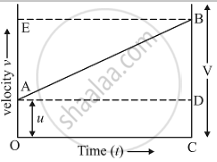# Show by Means of Graphical Method That: V = U + At, Where the Symbols Have Their Usual Meanings. - Science

Show by means of graphical method that: v = u + at, where the symbols have their usual meanings.

#### Solution

Consider the velocity-time graph of a body shown in figure.The body has an initial velocity (u)  at a point A and then, its velocity changes at a uniform rate from A to B in time, (t). In other words, there is a uniform acceleration (a) from A to B, and after time (t) its final velocity becomes (v) which is equal to BC in the graph. The time (t)  is represented by OC. To complete the figure, we draw the perpendicular CB from point C, and draw AD parallel to OC. BE is the perpendicular from point B to OE.

Now, initial velocity of the body, (u) = OA         ......(1)

And, final velocity of the body, (v) = BC         …… (2)
But from the graph BC = BD + DC
Therefore, v = BD + DC                                 ……(3)
Again DC = OA
So, v = BD + OA
Now, from equation (1),
So, v = BD + u                                                ......(4)
We should find out the value of the slope of a velocity-time graph which is equal to the acceleration,
Thus, Acceleration,
a = "BD"/"AD"
But AD = OC = t, so putting t in place of AD in the above relation, we get,
a = "BD"/t
So,  BD = at
Now, putting this value of BD in equation (4), we get the 1st equation of motion-:
v = u + at
where,
(a) - Acceleration
(v) - Final velocity
(u) - Initial velocity
(t) - Time taken

Concept: Velocity - Time Graphs
Is there an error in this question or solution?

#### APPEARS IN

Lakhmir Singh Science Class 9 Physics
Chapter 1 Motion
Long Answers | Q 38 | Page 42